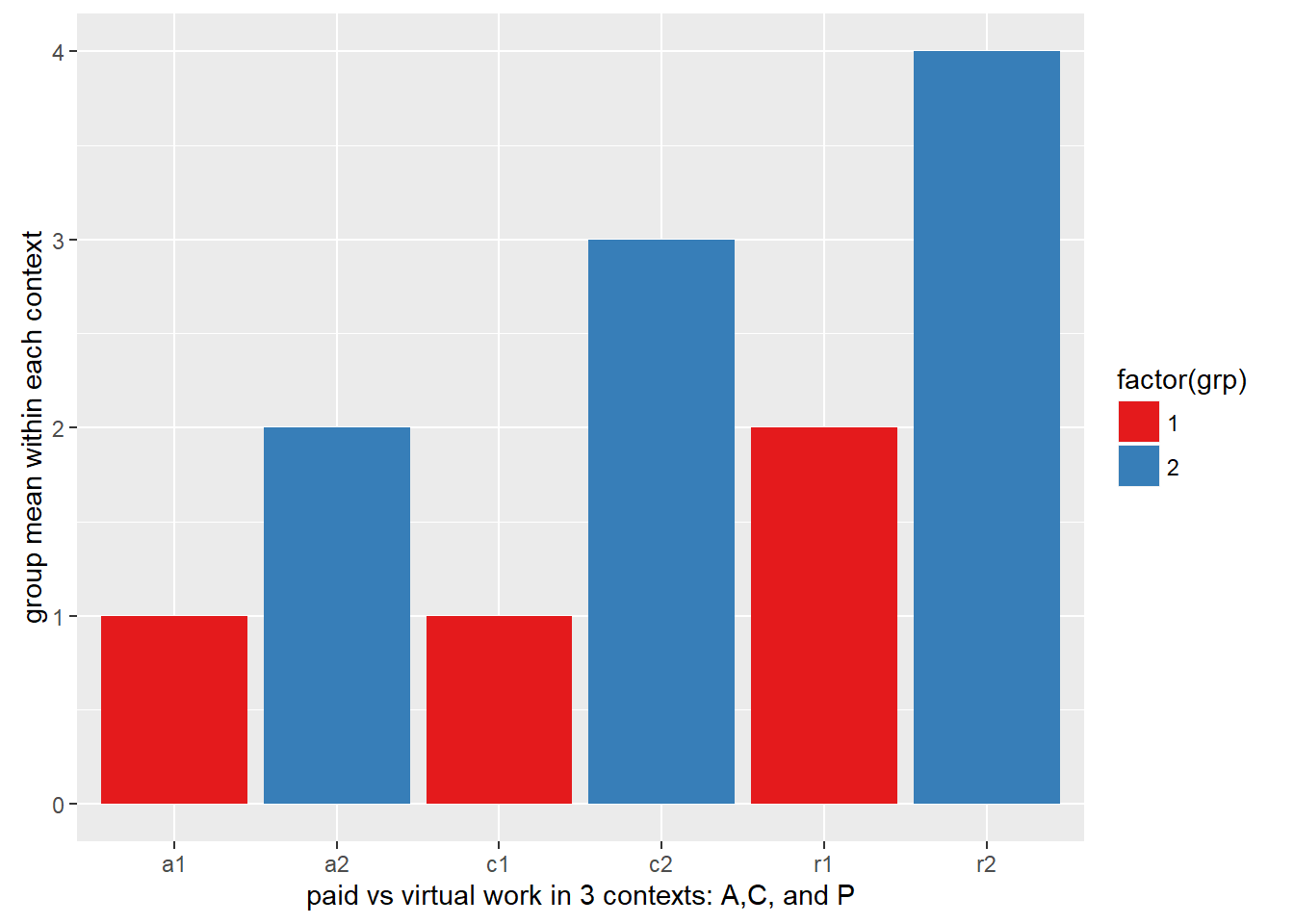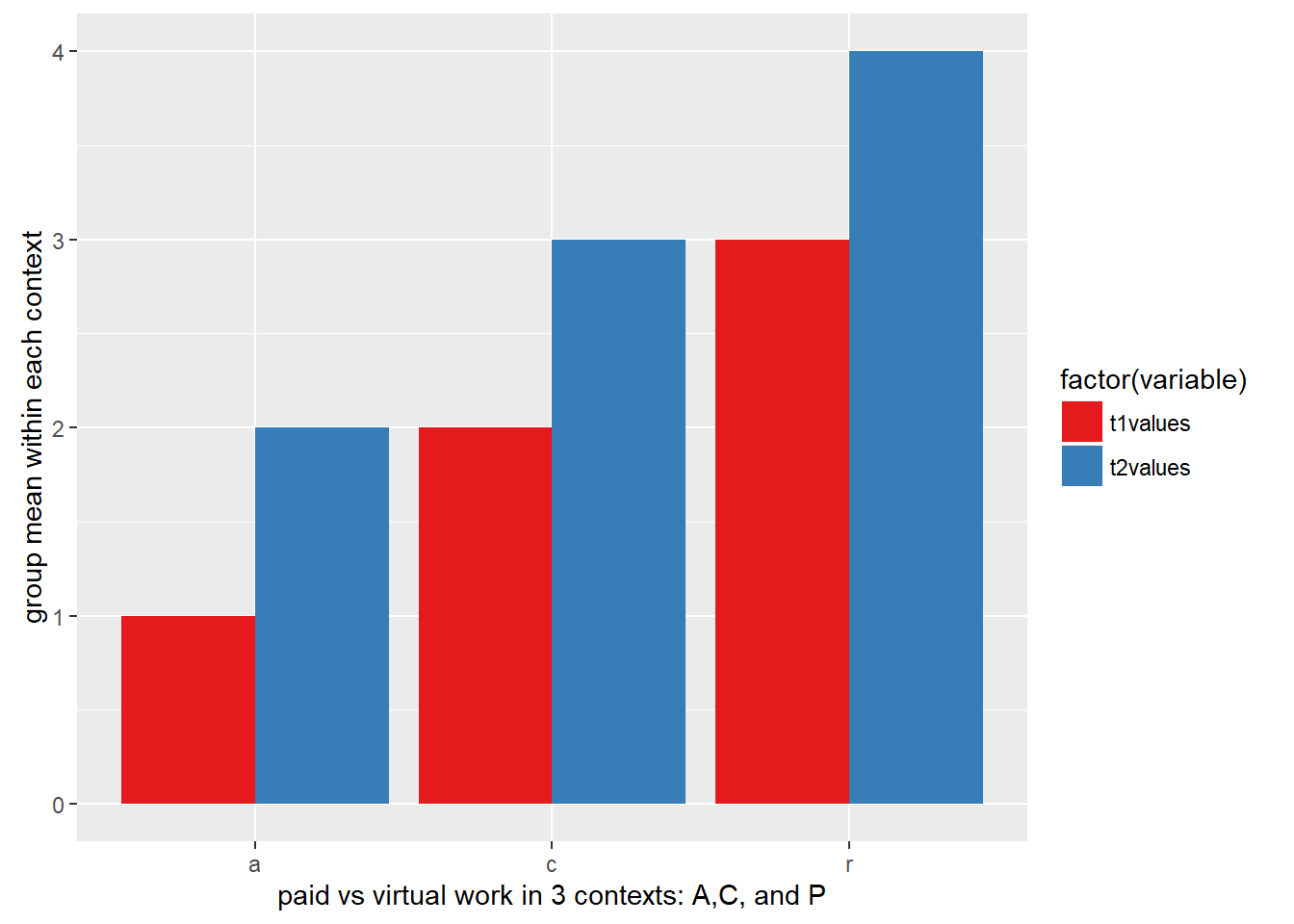#install.packages("rmarkdown", contriburl = "http://cran.r-project.org/src/contrib", type = "source")
library(rmarkdown)
library(ggplot2)

init data frame

df<- data.frame(columnname = c('a1','c1','r1','a2','c2','r2'),
values = c(1,1,2,2,3,4),
grp=c(1,1,1,2,2,2))

df
##   columnname values grp
## 1         a1      1   1
## 2         c1      1   1
## 3         r1      2   1
## 4         a2      2   2
## 5         c2      3   2
## 6         r2      4   2

data formatting long vs wide (for bargraph like the one below you need data in LONG format)

In the wide format, a subject’s repeated responses will be in a single row, and each response is in a separate column. A column for time 1, Time 2 etc. The row corresponds to all of one subjects data.

subject time1 time2 time3 age
Bill 12 14 18 24

In the long format, each row is one time point, so each subject has multiple rows.

subject trial time age
Bill 1 12 24
Bill 2 14 24
Bill 3 18 24

You’ll note that my dataframe above (named df) is already in LONG format, but lets say we had data in WIDE format and needed to convert it. You will need to group your data, in this case by the ‘columnname’ column.

#wide formatted data
df_wide<- data.frame(columnname = c('a','c','r'),
t1values = c(1,2,3),
t2values = c(2,3,4))

df_wide
##   columnname t1values t2values
## 1          a        1        2
## 2          c        2        3
## 3          r        3        4

to group your data you can use the ‘melt’ function in the ‘reshape2’ library in R. You specify what column should be unique and it pulls ____

library(reshape2)
df_wide_reshaped<-melt(df_wide,id =c("columnname"))
df_wide_reshaped
##   columnname variable value
## 1          a t1values     1
## 2          c t1values     2
## 3          r t1values     3
## 4          a t2values     2
## 5          c t2values     3
## 6          r t2values     4
creating the graph
• geom_bar=geometric bar (each bar in the graph); dodge = basically don’t put the bars on top of each other.
• scale_fill_brewer = choose color palette for each group (there are different combinations)

ggplot(df, aes(x=columnname, y=values, fill=factor(grp))) +
geom_bar(stat="identity", position="dodge") +
xlab("paid vs virtual work in 3 contexts: A,C, and P")+
ylab("group mean within each context")+
scale_fill_brewer(palette="Set1")#NOTE: The melt function changed our varaible names slightly
#NOTE: how close the bars are together in the two graphs, the one we reshaped has the bars pressed right up against each other while the original has space between every bar. This is because the column names in the column 'columnnames' in df_wide_reshaped matched exactly while they did not in 'df'
ggplot(df_wide_reshaped, aes(x=(columnname), y=value, fill=factor(variable))) +
geom_bar(stat="identity", position="dodge") +
xlab("paid vs virtual work in 3 contexts: A,C, and P")+
ylab("group mean within each context")+
scale_fill_brewer(palette="Set1")